Mathematics 3 And Surveying 3 October/November 2015 past paper

Exam Year:2015

This past paper applies to

MATHEMATICS III AND SURVEYING III
OCT./NOV.2015
Time: 3 hours

INSTRUCTIONS TO CANDIDATES

You should have the following for this examination.
Drawing instrument
Scientific calculator
This paper consists of Eight questions in TWO sections; A and B.
Answer FIVE questions choosing at least TWO questions from each section.
All questions carry equal marks.
Maximum marks for each part of a question are as shown.
Candidates should answer the questions in English.

SECTION A: MATHEMATICS III
Answer at least TWO questions from this section.
1. (a) Given matrixFind the values of  for which A is singular. (5 marks)

(b) Using the matrix inverse method, solve the following simultaneous equations:

3x + 4y – 6z = – 29
3x + 2y + 2z= 7
2x + 12z =50
(15 marks)

2. (a) Consider the data in table 1 and estimate f(4.12) using the Newton – Gregory difference interpolation polynomial.(10 marks)
(b) A probability density function is given by:Determine;
Value of k;
The expected value of x;
The probability that x is greater than 2.8.
(10 marks)

3. (a) The data in table 2 gives the corresponding values of x and y.Compute the pearson’s product moment coefficient of correlation.
Interpret your results in (i) above.
(10 marks)

(b) The data in table 3 shows the expenditure and income for Juhudi enterprisesDetermine the least squares regression equation of y on x.
(10 marks)

4. (a) Given that xn approximate root of the equation x3 – 3x + 3 = 0;

(i) Show using Newton – Raphson method that a better approximation xn+1 is given by:(ii) Find correct to four decimal places the root of the equation taking xo = -2. (10 marks)
(b) KK traders have a number of vehicles of which on average 3 are in use at any instant. Assuming that the number of vehicles in use at any instant follow a poisson distribution, find the probability that at any given instant:

(i) Not more than two vehicles are in use;
(ii) At least four vehicles are in use.
(7 marks)

(c) The years of experience of 100 surveyors are normally distributed with a mean of 18 years and standard deviation of 0.7 years. All possible samples of size 25 are drawn from this population and the means computed. Determine the mean and the standard error of the sampling distribution of the sample means.
(3 marks)

SECTION B: SURVEYING III
Answer at least TWO questions from this section.
5. (a) Table 4 shows observations taken from station T to locate points P, R, and S. Given the instrument constants are k = 100 and c = 0 compute:

(i) The horizontal distance TP, TR and TS;
(ii) The height differences between SP, SR and RP.
(18 marks)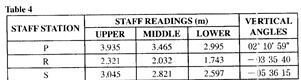(b) List two errors in stadia tacheometry.
(2 marks)
6. (a) Figure 1 shows height above a formation level taken at each grid intersection. Given the grid size 25 m X 25 m, compute the volume of the excavation.
(5 marks)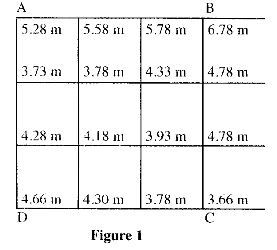(b) Table 5 shows volume in m3 at each chainage.
(i) Compute the aggregate volumes.
(ii) Plot the mass haul diagram to a vertical scale of 1 cm to 200 m3 and horizontal scale of 1 cm to 50 m.
(15 marks)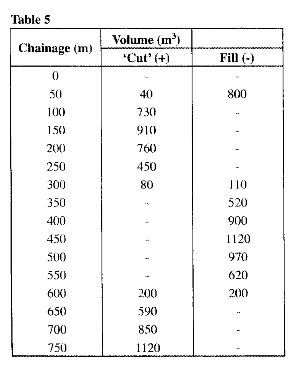7. (a) Using illustrations where appropriate, outline three methods used to check verticality of multistory buildings.
(9 marks)

(b) Calculate the area of the plot defined by the data given in table 6 using:
(i) Trapezoidal;
(ii) Simpson’s;
(iii) Comment on the results.
(11 marks)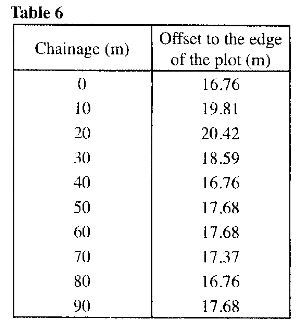8. (a) Table 7 shows coordinates of images of points, S and T as they appear on a vertical photograph. Given the following information:

Camera focal length = 220 mm
Altitude of S = 450 m
Altitude of T = 750 m
Altitude of aircraft = 3000 m

Determine the ground distance ST.
(10 marks)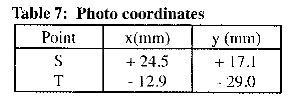(b) Illustrate the operating principle of a mirror stereoscope.
(10 marks)